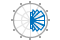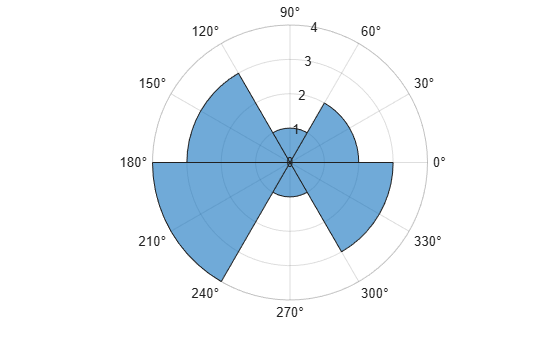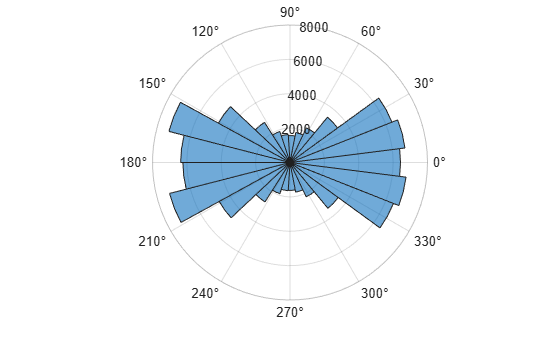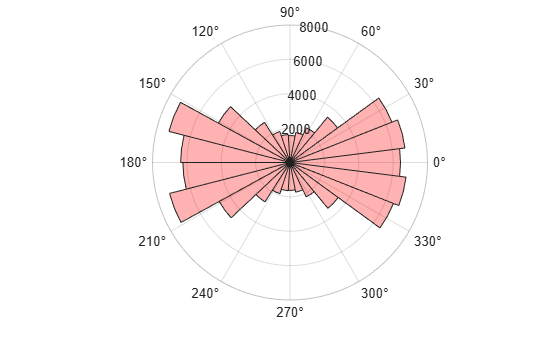# polarhistogram

Histogram chart in polar coordinates

•## Syntax

``polarhistogram(theta)``
``polarhistogram(theta,nbins)``
``polarhistogram(theta,edges)``
``polarhistogram('BinEdges',edges,'BinCounts',counts)``
``polarhistogram(___,Name,Value)``
``polarhistogram(pax,___)``
``h = polarhistogram(___)``

## Description

example

````polarhistogram(theta)` creates a histogram plot in polar coordinates by sorting the values in `theta` into equally spaced bins. Specify the values in radians.```

example

````polarhistogram(theta,nbins)` uses the number of bins specified by the positive integer, `nbins`.```
````polarhistogram(theta,edges)` sorts `theta` into bins with bin edges specified by the vector, `edges`. All bins include the left edge, but only the last bin includes the right edge. In other words, the last bin includes both edges.```
````polarhistogram('BinEdges',edges,'BinCounts',counts)` uses the manually specified bin edges and associated bin counts. The `polarhistogram` function does not do any data binning.```

example

````polarhistogram(___,Name,Value)` specifies additional options using one or more name-value pair arguments. For example, you can use semitransparent bars by specifying `'FaceAlpha'` and a scalar value between 0 and 1.```
````polarhistogram(pax,___)` plots into the polar axes specified by `pax` instead of into the current axes.```

example

````h = polarhistogram(___)` returns the `Histogram` object. Use `h` to modify the histogram after it is created. For a list of properties, see Histogram Properties.```

## Examples

collapse all

Create a vector of values between 0 and $2\pi$. Create a histogram chart that shows the data sorted into six bins.

```theta = [0.1 1.1 5.4 3.4 2.3 4.5 3.2 3.4 5.6 2.3 2.1 3.5 0.6 6.1]; polarhistogram(theta,6)```Before R2022a, polar axes do not include degree symbols by default. To add them, get the polar axes using `pax = gca`. Then modify the tick labels using `pax.ThetaTickLabel = string(pax.ThetaTickLabel) + char(176)`.

Create a histogram plot from 100,000 values between $-\pi$ and $\pi$, and sort the data into 25 bins.

```theta = atan2(rand(100000,1)-0.5,2*(rand(100000,1)-0.5)); polarhistogram(theta,25);```Create a histogram chart in polar coordinates, and then change its appearance. Specify the bar colors by setting the `FaceColor` property to a character vector of a color name, such as `'red'`, or an RGB triplet. Specify the transparency by setting the `FaceAlpha` property to a value between 0 and 1.

```theta = atan2(rand(100000,1)-0.5,2*(rand(100000,1)-0.5)); polarhistogram(theta,25,'FaceColor','red','FaceAlpha',.3);```Create a histogram chart in polar coordinates. Assign the histogram object to the variable `h`.

```theta = atan2(rand(100000,1)-0.5,2*(rand(100000,1)-0.5)); h = polarhistogram(theta,25)``````h = Histogram with properties: Data: [100000x1 double] Values: [6232 7236 4699 2717 1908 1641 1622 1755 2207 3463 6471 6806 6429 6741 6380 3422 2233 1777 1570 1633 1899 2768 4755 7273 6363] NumBins: 25 BinEdges: [-3.1416 -2.8903 -2.6389 -2.3876 -2.1363 -1.8850 -1.6336 -1.3823 -1.1310 -0.8796 -0.6283 -0.3770 -0.1257 0.1257 0.3770 0.6283 0.8796 1.1310 1.3823 1.6336 1.8850 2.1363 2.3876 2.6389 2.8903 3.1416] BinWidth: 0.2513 BinLimits: [-3.1416 3.1416] Normalization: 'count' FaceColor: 'auto' EdgeColor: [0 0 0] Use GET to show all properties ```

Use `h` to access and modify properties of the histogram object after it is created. For example, show just the histogram outline by setting the `DisplayStyle` property of the histogram object.

`h.DisplayStyle = 'stairs';`## Input Arguments

collapse all

Data to distribute among bins, specified as a vector or a matrix. `polarhistogram` creates one histogram, regardless of whether you specify a vector or a matrix. Specify the values in radians. To convert degrees to radians, use `deg2rad`.

Values that correspond to the same angle direction differ by exactly 2π, and are sorted into the same bin. `polarhistogram` does not include `NaN`, `Inf`, and `-Inf` values in any bin.

Example: `theta = [0 0.4 0.5 0.7 2.3 3.0 1.7 0.3];`

Data Types: `single` | `double` | `int8` | `int16` | `int32` | `int64` | `uint8` | `uint16` | `uint32` | `uint64`

Number of bins, specified as a positive integer. If you do not specify `nbins`, then `polarhistogram` automatically calculates how many bins to use based on the values in `theta`.

Example: `nbins = 15;`

Bin edges, specified as a vector. The difference between the minimum and maximum edge values must be less than or equal to 2π.

Example: ```polarhistogram('BinEdges',[0 pi/3 pi 3*pi/2 2*pi],'BinCounts',[5 3 4 6])```

Bin counts, specified as a vector. Use this option if you perform the bin counts calculation separately and you do not want `polarhistogram` to do any data binning.

Example: ```polarhistogram('BinEdges',[0 pi/3 pi 3*pi/2 2*pi],'BinCounts',[5 3 4 6])```

`PolarAxes` object. If you do not specify the polar axes, then `polarhistogram` uses the current axes. `polarhistogram` does not support plotting into Cartesian axes.

### Name-Value Arguments

Specify optional pairs of arguments as `Name1=Value1,...,NameN=ValueN`, where `Name` is the argument name and `Value` is the corresponding value. Name-value arguments must appear after other arguments, but the order of the pairs does not matter.

Before R2021a, use commas to separate each name and value, and enclose `Name` in quotes.

Example: `polarhistogram(theta,'FaceAlpha',0.3)` creates a histogram chart with semitransparent bars.

The histogram properties listed here are only a subset. For a complete list, see Histogram Properties.

Bin limits, specified as a two-element vector of the form `[bmin,bmax]`, where `bmin` is less than `bmax`.

This option plots a histogram using the input array values that fall between `bmin` and `bmax` inclusive. That is, `theta(theta>=bmin & theta<=bmax)`.

Example: `polarhistogram(theta,'BinLimits',[-pi/2 pi/2])` plots a histogram using only the values in `theta` that are between `-pi/2` and `pi/2` inclusive.

Width across the top of the bins, specified as a scalar less than 2π.

`polarhistogram` uses a maximum of 65,536 bins (or 216). If the specified bin width requires more bins, then `polarhistogram` uses the maximum number of bins and adjust the bin width accordingly.

Example: `polarhistogram(theta,'BinWidth',pi)` uses bins with a width of π.

Type of normalization, specified as one of the values in this table.

ValueDescription
`'count'`

Default normalization scheme. The height of each bar is the number of observations in each bin. The sum of the bar heights is `numel(theta)`.

`'probability'`

The height of each bar is the relative number of observations. Each height is calculated as (number of observations in bin/total number of observations). The sum of the bar heights is `1`.

`'countdensity'`

The height of each bar is the number of observations in bin/width of bin.

`'pdf'`

Probability density function estimate. The height of each bar is (number of observations in the bin)/(total number of observations * width of bin). The area of each bar is the relative number of observations. The sum of the bar areas is `1`.

`'cumcount'`

The height of each bar is the cumulative number of observations in each bin and all previous bins. The height of the last bar is `numel(theta)`.

`'cdf'`

Cumulative distribution function estimate. The height of each bar is equal to the cumulative relative number of observations in the bin and all previous bins. The height of the last bar is `1`.

Example: `polarhistogram(theta,'Normalization','pdf')` plots an estimate of the probability density function for `theta`.

Histogram display style, specified as one of these values:

• `'stairs'` — Display the histogram outline only.

• `'bar'` — Show each individual bar with a filled interior.

Example: `polarhistogram(theta,'DisplayStyle','stairs')` plots the outline of the histogram.

Transparency of histogram bars, specified as a scalar value between `0` and `1` inclusive. `polarhistogram` uses the same transparency for all the bars of the histogram. A value of `1` means fully opaque and `0` means completely transparent (invisible).

Example: `polarhistogram(theta,'FaceAlpha',.5)` creates a histogram plot with semi-transparent bars.

Histogram bar color, specified as `'auto'`, an RGB triplet, a hexadecimal color code, a color name, or a short name. The default value of `'auto'` lets the histogram choose the color.

For a custom color, specify an RGB triplet or a hexadecimal color code.

• An RGB triplet is a three-element row vector whose elements specify the intensities of the red, green, and blue components of the color. The intensities must be in the range `[0,1]`, for example, ```[0.4 0.6 0.7]```.

• A hexadecimal color code is a string scalar or character vector that starts with a hash symbol (`#`) followed by three or six hexadecimal digits, which can range from `0` to `F`. The values are not case sensitive. Therefore, the color codes `"#FF8800"`, `"#ff8800"`, `"#F80"`, and `"#f80"` are equivalent.

Alternatively, you can specify some common colors by name. This table lists the named color options, the equivalent RGB triplets, and hexadecimal color codes.

Color NameShort NameRGB TripletHexadecimal Color CodeAppearance
`"red"``"r"``[1 0 0]``"#FF0000"``"green"``"g"``[0 1 0]``"#00FF00"``"blue"``"b"``[0 0 1]``"#0000FF"``"cyan"` `"c"``[0 1 1]``"#00FFFF"``"magenta"``"m"``[1 0 1]``"#FF00FF"``"yellow"``"y"``[1 1 0]``"#FFFF00"``"black"``"k"``[0 0 0]``"#000000"``"white"``"w"``[1 1 1]``"#FFFFFF"``"none"`Not applicableNot applicableNot applicableNo color

Here are the RGB triplets and hexadecimal color codes for the default colors MATLAB® uses in many types of plots.

`[0 0.4470 0.7410]``"#0072BD"``[0.8500 0.3250 0.0980]``"#D95319"``[0.9290 0.6940 0.1250]``"#EDB120"``[0.4940 0.1840 0.5560]``"#7E2F8E"``[0.4660 0.6740 0.1880]``"#77AC30"``[0.3010 0.7450 0.9330]``"#4DBEEE"``[0.6350 0.0780 0.1840]``"#A2142F"`If you specify `DisplayStyle` as `'stairs'`, then `polarhistogram` does not use the `FaceColor` property.

Example: `polarhistogram(theta,'FaceColor','g')` creates a histogram plot with green bars.

## Limitations

• `polarhistogram` does not support creating histograms of categorical data in polar axes.

## Version History

Introduced in R2016b